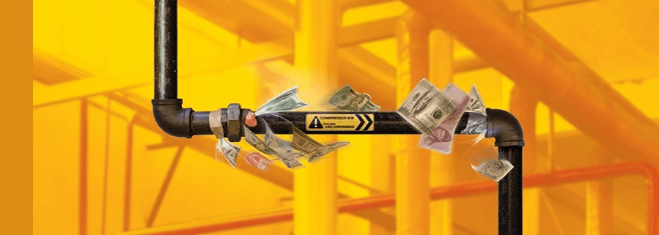## Estimating the Cost of Compressed Air Systems LeaksLeaks in a compressed air system can waste thousands of dollars of electricity per year. In fact, in many plants, the leakage can account for up to 30% of the total operational cost of the compressor. Some of the most common areas where you might find a leak would be at connection joints like valves, unions, couplings, fittings, etc. This not only wastes energy but it can also cause the compressed air system to lose pressure which reduces the end use product’s performance, like an air operated actuator being unable to close a valve, for instance.

One way to estimate how much leakage a system has is to turn off all of the point-of-use devices / pneumatic tools, then start the compressor and record the average time it takes for the compressor to cycle on and off. The total percentage of leakage can be calculated as follows:

Percentage = [(T x 100) / (T + t)]

T = on time in minutes
t = off time in minutes

The percentage of compressor capacity that is lost should be under 10% for a system that is properly maintained.

Another method to calculate the amount of leakage in a system is by using a downstream pressure gauge from a receiver tank. You would need to know the total volume in the system at this point though to accurately estimate the leakage. As the compressor starts to cycle on,  you want to allow the system to reach the nominal operating pressure for the process and record the length of time it takes for the pressure to drop to a lower level. As stated above, any leakage more than 10% shows that improvements could be made in the system.

Formula:

(V x (P1 – P2) / T x 14.7) x 1.25

V= Volumetric Flow (CFM)
P1 = Operating Pressure (PSIG)
P2 =  Lower Pressure (PSIG)
T = Time (minutes)
14.7 = Atmospheric Pressure
1.25 = correction factor to figure the amount of leakage as the pressure drops in the system

Now that we’ve covered how to estimate the amount of leakage there might be in a system, we can now look at the cost of a leak. For this example, we will consider a leak point to be the equivalent to a 1/16″ diameter hole.

A 1/16″ diameter hole is going to flow close to 3.8 SCFM @ 80 PSIG supply pressure. An industrial sized air compressor uses about 1 horsepower of energy to make roughly 4 SCFM of compressed air. Many plants know their actual energy costs but if not, a reasonable average to use is \$0.25/1,000 SCF generated.

Calculation :

3.8 SCFM (consumed) x 60 minutes x \$ 0.25 divided by 1,000 SCF

= \$ 0.06 per hour
= \$ 0.48 per 8 hour work shift
= \$ 2.40 per 5-day work week
= \$ 124.80 per year (based on 52 weeks)

As you can see, that’s a lot of money and energy being lost to just one small leak. More than likely, this wouldn’t be the only leak in the system so it wouldn’t take long for the cost to quickly add up for several leaks of this size.

If you’d like to discuss how EXAIR products can help identify and locate costly leaks in your compressed air system, please contact one of our application engineers at 800-903-9247.

Justin Nicholl
Application Engineer
justinnicholl@exair.com
@EXAIR_JN